Combine Multiple Worksheets into One

In this article, we are going to learn how to assemble the data from different workbooks through VBA in Microsoft Excel.

Let’s understand with a simple exercise, how to merge spread sheet through VBA in Microsoft Excel.

We have 3 different Excel files. 2 files are to xlsx and one file is to xlsm in a folder. We want to collate both data sets in Excel file to keep few things in mind that in every data, rows and columns can be increase and decrease, and also we don’t know the Excel file names and how many files are there in a folder.

In this sheet, we want to combine the data:-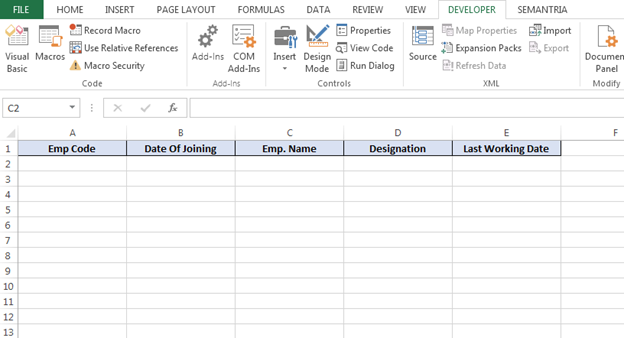1st Data:-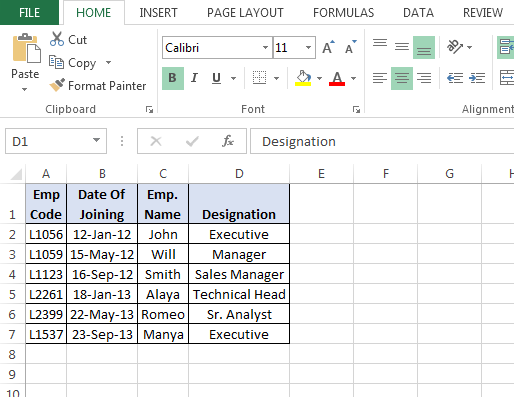2nd Data:-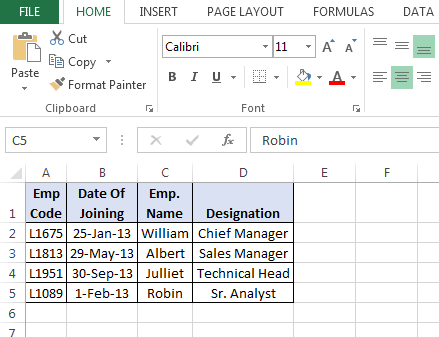3rd Data:-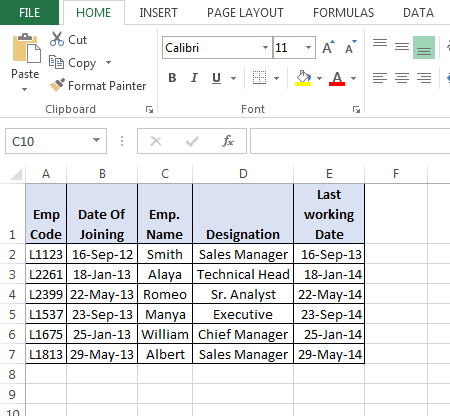Now, we want to collate the data in a sheet. We need to follow below given steps and code:-

• Press the key Alt+F11 to open the VBE page to write the macro.
• Then go to insert tab and insert a module.
• Write below mentioned code in the page.

Sub Collate_Data()

Dim FolderpathAs String, filePath As String, Filename As String

Folderpath = “E:\Excel Tips\New VBA topics\HR Data\”      ‘(mention the file path)

filePath = Folderpath& “*xls*”

Filename = Dir(filePath)

Dim LastrowAs Long, Lastcolumn As Long
Do While Filename <> “”

Workbooks.Open (Folderpath& Filename)

Lastrow = ActiveSheet.Cells(Rows.count, 1).End(xlUp).Row

Lastcolumn = ActiveSheet.Cells(1, Columns.count).End(xlToLeft).Column

Range(Cells(2, 1), Cells(Lastrow, Lastcolumn)).Copy

ActiveWorkbook.Close

erow = Sheet1.Cells(Rows.count, 1).End(xlUp).Offset(1, 0).Row

ActiveSheet.Paste Destination:=Worksheets(“Sheet1″).Range(Cells(erow, 1), Cells(erow, 5))

Filename = Dir

Loop

End Sub

Code explanation: – Firstly, we will define the all variable which we need to use while describing the code. Then we will use Do While loop in code to run the program for all Excel files. Then we will define the path of files, and after that we will define the last row and column in Excel sheet and then we will define the destination to paste the data.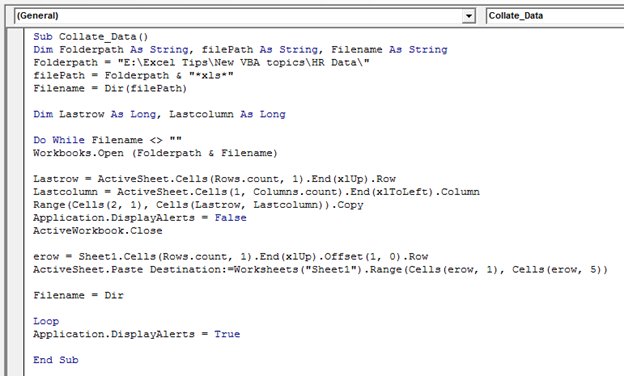• To run the macro, press the key F5.
• Data of both sheets will be copied and pasted in an Excel sheet.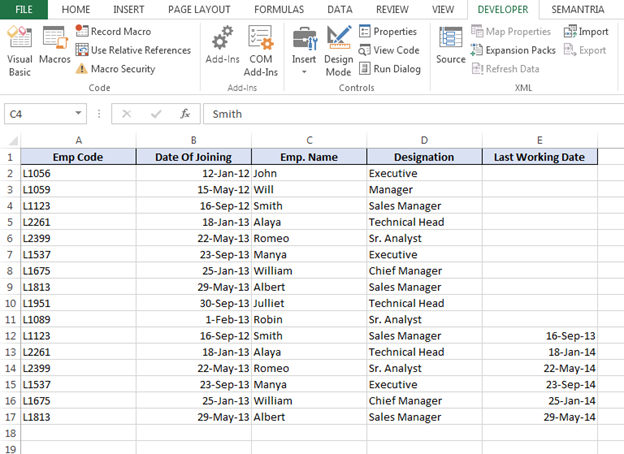This is the way we can collate the data in a sheet from different files of Excel through VBA in Microsoft Excel.

Terms and Conditions of use

The applications/code on this site are distributed as is and without warranties or liability. In no event shall the owner of the copyrights, or the authors of the applications/code be liable for any loss of profit, any problems or any damage resulting from the use or evaluation of the applications/code.